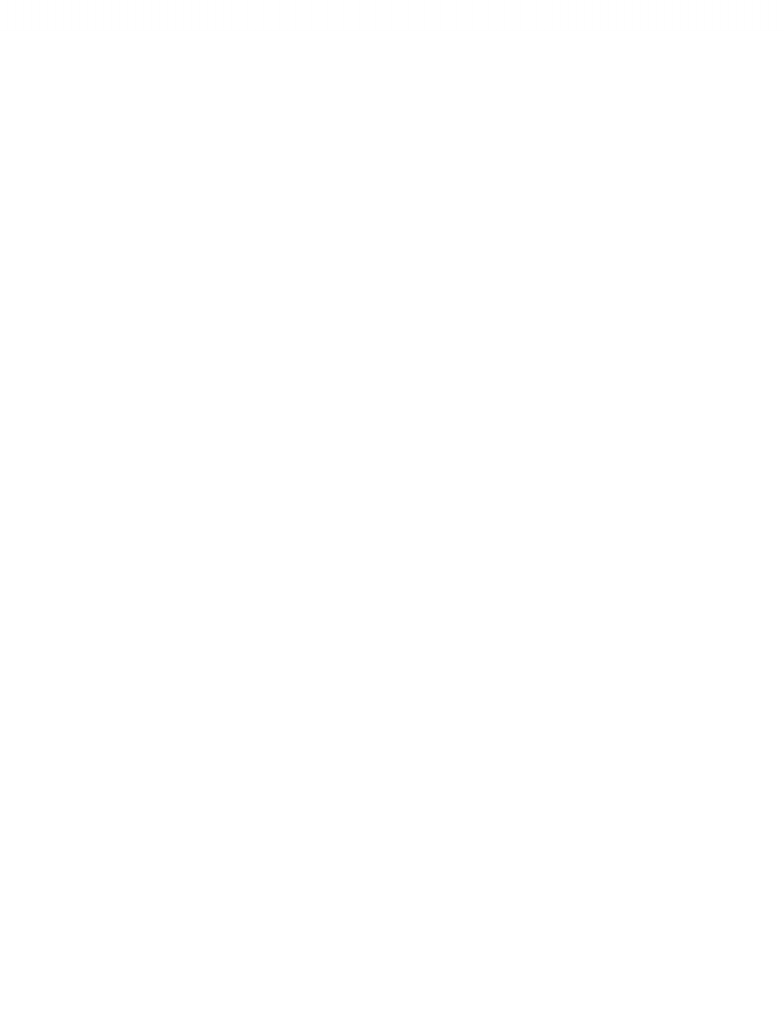# Cost Behaviour

23 views1 pages
School
Ryerson University
Department
Accounting
Course
ACC 406
ProfessorHomework: P3-41
Chapter 3 - Cost Behaviour
Cost Behaviour is the relationship between cost and the activity volume.
Variable Cost + Fixed Cost = Total Cost
High-low Method
1.Find Highest and Lowest point
2.Variable cost per unit > V= YH YL / XH - XL
3.Fixed cost > F = Y-(V)(X) [Either use High or Low]
4.Y = F (V)(x)
Scatter plot Method
Method of least Squares
.:. Estimate the cost function/equation/formula
Total Cost = Ttl Fixed Cost + (Variable Cost per unit)(Activity Volume)
Y = F + (v)(x)
www.notesolution.com
Unlock document

This preview shows half of the first page of the document.
Unlock all 1 pages and 3 million more documents.